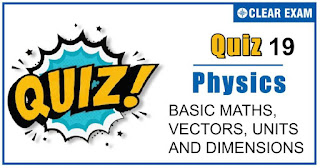## UNITS AND MEASUREMENTS-QUIZ 19

As per analysis for previous years, it has been observed that students preparing for NEET find Physics out of all the sections to be complex to handle and the majority of them are not able to comprehend the reason behind it. This problem arises especially because these aspirants appearing for the examination are more inclined to have a keen interest in Biology due to their medical background. Furthermore, sections such as Physics are dominantly based on theories, laws, numerical in comparison to a section of Biology which is more of fact-based, life sciences, and includes substantial explanations. By using the table given below, you easily and directly access to the topics and respective links of MCQs. Moreover, to make learning smooth and efficient, all the questions come with their supportive solutions to make utilization of time even more productive. Students will be covered for all their studies as the topics are available from basics to even the most advanced. .

Q1. The dimensions of permittivity ε0 areThe dimensions of permittivity ε0 are
•  A2 T2 M-1 L-3
•  A2 T4 M-1 L-3
•  A-2 T-4 ML3
•  A2 T-4 M-1 L-3
Solution
(b) F=1/(4πε0 ) (q1 q2)/r2
⇒ ε0=(|q1 ||q2 |)/([F][r2])=[A2 T2 ]/([MLT-2 ][L2])=[A2 T4 M-1 L-3]

Q2.Which one of the following is not a unit of Young’s modulus?
•  Nm-1
•  Nm-2
•  Dyne cm-2
•  Mega pascal
Solution
(a) Young’s modulus Y=stress/strain=N/m2 or pascal (in SI system)
And Y=dyne/cm2 (in CGS system)
Then, Nm(-1) is not the unit of Young’s modulus.

Q3. The unit of surface tension in SI system is
•   Dyne/cm2
•  Newton /m
•  Dyne/cm
•  Newton/m2
Solution
(b) Surface tension=Force/Length=newton/metre

Q4. The speed (v) of ripples on the surface of water depends on surface tension (σ), density (ρ) and wavelength (λ). The square of speed (v) is proportional to
•  σ/ρλ
•  ρ/σλ
•  λ/σρ
•  Ρλσ
Solution
(a) Let v∝σa ρb λc Equating dimensions on both sides.
[M0 LT-1 ]∝[MT-2 ]a [ML-3 ]b [L]c
∝[M](a+b) [L](-3b+c) [T](-2a)
Equating the powers of M, L, T on both sides, we get a+b=0 -3b+c=1 -2a=-1
Solving, we get a=1/2,b=-1/2,c=-1/2
∴ v∝σ(1/2) ρ(-1/2) λ(-1/2)
∴ v2∝σ/ρλ

Q5.The dimension of 1/2 ϵ0 E2, where ϵ0 is permittivity of free space and E is electric field, is
•  MLT1
•  ML2 T-2
•  ML-1 T-2
•  ML2 T-1
Solution
(c) [1/2 ∈0 E2 ]= [Energy density]
=(ML2 T-2/L3 =ML-1 T-2

Q6. If the dimensions of a physical quantity are given by Ma Lb Tc, then the physical quantity will be
•  Pressure if a =1,b =-1,c =-2
•  Velocity if a = 1,b = 0,c =-1
• Acceleration if a =1,b = 1,c=-2
•  Force if a = 0,b = -1,c =-2
Solution
(a)Pressure if a =1,b =-1,c =-2

Q7.The dimensional formula of modulus of rigidity is
•  [ML-2 T-2 ]
•  [ML-3 T2 ]
•  [ML2 T-2 ]
•  [ML-1 T-2 ]
Solution
(d) Modulus of rigidity η=(F/A)/ϕ Dimensions of η=[MLT-2 ]/[L2 ]
=[ML-1 T-2]

Q8.SI unit of pressure is
•  Pascal
•  dynes/cm2
•  cm of Hg
•  Atmosphere
Solution
(a)Pascal

Q9.The dimensional formula of capacitance in terms of M,L,T and I is
•  [ML2 T2 I2]
•  [ML-2 T4 I2]
•  [M-1 L3 T3 I]
•  [M-1 L-2 T4 I2]
Solution
(d) Capacitances C=Q/V=QQ/W=Q2/W=(I2 t2)/W,
[C]=([I2 T2])/([ML2 T-2])=[M-1 L-2 T4 I2]

Q10. Which of the following groups have different dimensions
•  Potential difference, EMF, voltage
•  Pressure, stress, young’s modulus
•  Heat , energy, work-done
•  Dipole moment, electric flux, electric field
Solution
(d)Dipole moment, electric flux, electric field## Want to know more

Please fill in the details below:

## Latest NEET Articles\$type=three\$c=3\$author=hide\$comment=hide\$rm=hide\$date=hide\$snippet=hide

Name

ltr
item
BEST NEET COACHING CENTER | BEST IIT JEE COACHING INSTITUTE | BEST NEET, IIT JEE COACHING INSTITUTE: UNITS AND MEASUREMENTS-QUIZ 19
UNITS AND MEASUREMENTS-QUIZ 19